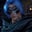Related Tags

julia
communitycreator
rationalnumbers
complexnumbers

# What are complex and rational numbers in Julia?Gutha Vamsi Krishna

In this shot, we will learn about complex and rational numbers in Julia.

### Complex numbers

A complex number is a number that can be expressed in the form of a+bi, where a and b are real numbers and i is the imaginary part, meaning that i is $\sqrt{-1}$.

In Julia, we represent a complex number as a+bim, where a and b are real numbers and im is the imaginary part.

### Syntax

We can create a complex number in Julia using two ways:

1. Declaring it in the following way:
y = a+bim


where a and b are real numbers and im is the imaginary part.

1. Using the complex method, which accepts real numbers as parameters (a, b in the code below) and returns a complex number.
complex(a,b)


Let’s look at an example of this.

### Example

#declaring complex number using normal way
x = 3+4im
println(x)

#declaring complex number usign Complex
y = complex(4, 5)
println(y)

### real() and imag() functions

We can get the real and imaginary parts of a complex number using the real() and imag() functions respectively.

### Example

Let’s take a look at the following code snippet to understand it better.

#declaring complex number using normal way
x = 3+4im

# get real part from complex number
r = real(x)
println("Real part of x is $r") # get imaginary part from complex number i = imag(x) println("Imaginary part of x is$i")

### Rational numbers

A rational number is any number that can be expressed as the fraction p/q of two integers.

In Julia, we represent a rational number in the p//q format.

Let’s take a look at an example.

### Example

#declaring a rational number
y = 2//3
println(y)


We use rational numbers in Julia where we feel that float numbers cannot be used. For example, dividing 1 with 3 returns 0.3333333333333333 .... When we don’t want to use this kind of result, then we can directly use the representation 1//3 for the rational number 1/3.

#representing float number
x = 1/3
println(x)

#representing rational number
y = 1//3
println(y)

### num() and den() functions

We can get the numerator and denominator of a rational number using num() and den(), respectively.

Let’s take a look at the following code snippet where we get the numerator and denominator from a rational number.

### Example

#declaring rational number
y = 1//3

#display the numerator of a rational number
println(numerator(y))

#display the denominator of a rational number
println(denominator(y))

RELATED TAGS

julia
communitycreator
rationalnumbers
complexnumbers

CONTRIBUTORGutha Vamsi Krishna
RELATED COURSES

View all Courses

Keep Exploring

Learn in-demand tech skills in half the time